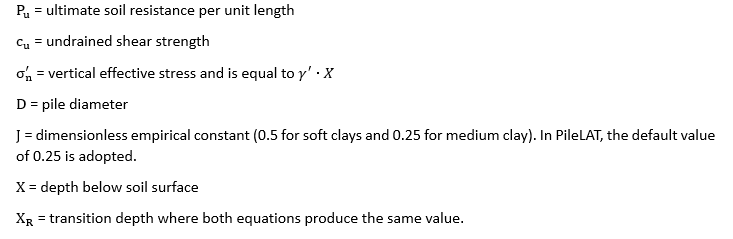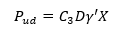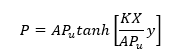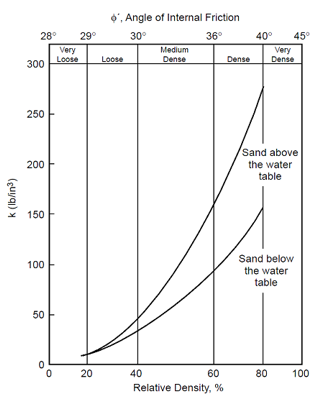top of page

# API p-y curve models for laterally loaded piles

A wide range of p-y curve models are available in our PileLAT and PileGroup programs for modeling the behavior of soils and rocks under lateral loading. This blog presents and discusses API p-y curve models for laterally loaded piles.

API model for soft clay

Soft clay can be modeled by the method recommended in API RP 2GEO 1st Edition (2014), where the ultimate resistance (Pu) of soft clay is determined in the same way as Matlock (1970).where:The only difference between API model and Matlock (1970)'s model is that the piece-wise curves are used as shown in the figures below for both static and cyclic loading conditions.Figure 1 P-Y curve for soft clay (API) model under static loading conditionFigure 2 P-Y curve for soft clay (API) model under cyclic loading condition

The following table tabulates the lateral soil resistance-deflection relationship for short-term static loading based on API RP 2GEO 1st Edition (2014).The reference displacement yc is calculated by the equation below:where E50 is the strain at one-half the maximum stress for an undrained tri-axial compression test and is based on the recommendations in the following table.Note that there some differences in the p-y curves for soft clay between API RP2A (2002) and API RP 2GEO (2014). The following figure shows the different p-y curves under the short-term static loading for (1) p-y curve for soft clay based on API (2002), (2) p-y curve for soft clay based on API RP 2GEO (2014) and (3) Matlock Soft Clay.Figure 3 P-Y curve comparison for soft clay under short-term static loading

Our PileLAT program has two options for API Clay models: (1) API Clay RP2A and (2) API Clay RP2GEO. The following figure shows the default P-Y parameter input for API Clay RP2A model based on API RP2A (2002) in PileLAT.Figure 4 P-Y parameter input dialog for API Clay model in PileLATFigure 5 Examples of P-Y curves for soft clay (API) model in PileLAT

API model for sand

For API sand model (API 2014), the ultimate lateral bearing capacity for sand at shallow depth is calculated as:The ultimate lateral bearing capacity for sand at deep depth is calculated as:whereAt a given depth (X), the smaller value from the above two equations should be used as the ultimate lateral bearing capacity.

P-Y curves for API Sand under both static and cyclic loading conditions are shown in the figure below.Figure 6 P-Y curve for API Sand model under both static and cyclic loading conditions

The lateral soil resistance - deflection (p-y) relationship is described by the following equation:where

P is actual lateral resistance

X is the depth below the ground surface

K is the initial modulus of subgrade reaction determined from Figure 6.8.7-1 of the API RP 2GEO 1st Edition (2014)

y is lateral deflectionFigure 7 Variation of C1, C2 and C3 with the friction angle for API sand model (after API 2014)Figure 8 Variation of initial modulus of subgrade with the friction angle for API sand model (after API 2014)

The following figure shows the default P-Y parameter input for API Sand model in PileLAT.Figure 9 P-Y parameter input dialog for the API Sand in PileLATFigure 10 Examples of P-Y curves for sand (API) model in PileLAT

10,250 views